#Vector Representations of SolutionsConsider the linear system of differential equations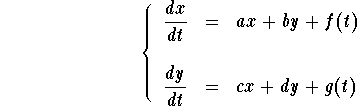This system may be rewritten using matrix-notation. Indeed, set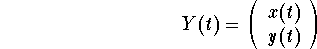,

then the above system is equivalent to the matricial equation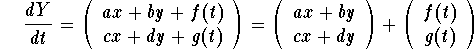.

Using the matrix product, we get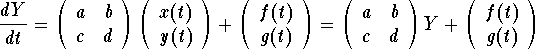.

The matrix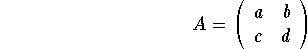is called the coefficient matrix of the system. Note that the coefficients of the matrix A can be constant or not. The vector function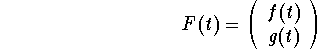is called the nonhomogeneous term.

Remark: One may think that the equation above is only valid for linear systems of two equations. However, that is not the case. For example, consider the linear system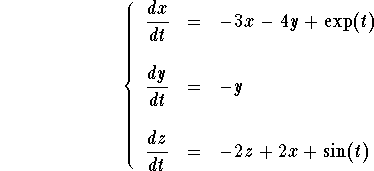Then, in matricial notation, the system is equivalent to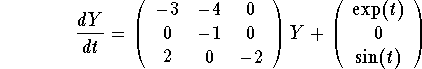,

where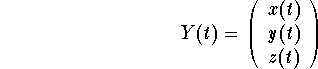.

## Equilibrium Points of Homogeneous Linear Systems

Consider the homogeneous linear system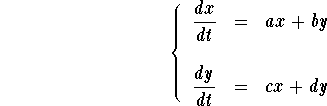The equilibrium points are given by the equations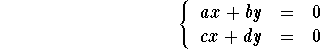Clearly, x=0 and y=0 give a trivial solution. Hence, the function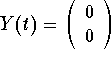gives a constant solution to the linear system. We call it the trivial solution. In general, the equilibrium points are the intersection between two lines. Since the two lines intersect, they are the same (if parallel) or the intersection is reduced to one point. So, the set of equilibrium points is the entire line ax+by=0, or the trivial point (0,0). This conclusion is related to the determinant of the matrix coefficient. Indeed, if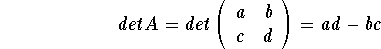is not equal to 0 (zero), then we have one equilibrium point (the trivial one).

## The Linearity Principle

This is may be the most important property for linear systems. Consider the homogeneous linear system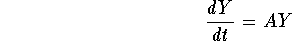,

then

1.
if Y(t) is a solution and k is a constant, then k Y(t) is also a solution;
2.
if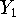and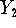are two solutions, then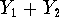is also a solution.

This clearly implies that ifandare two solutions and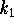and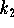are two arbitrary constants, then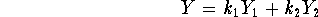is also a solution. This conclusion is also known as the Principle of Superposition.

Clearly, from the Principle of Superposition, we may generate plenty of solutions once two solutions are known. The natural question to ask therefore, is whether we have obtained all the solutions. In order to better appreciate this problem let's consider the following example.

Example: Consider the linear system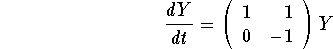Show that any solution Y to this system is given as,

where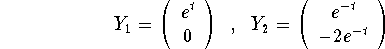,

andandare two constants.

Answer: It is easy to check that indeedandare solutions to the given system. Let Y be any solution. Set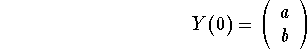.

By the uniqueness and existence theorem, Y is the only solution to the IVP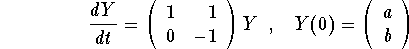.

Let us findandsuch that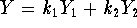. If this is the case, we should have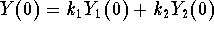, which gives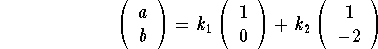,

which implies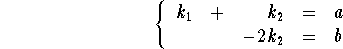Clearly, this gives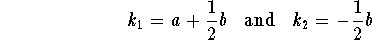.

Consider the function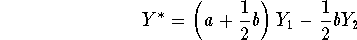.

The linearity principle implies that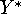is a solution. And, since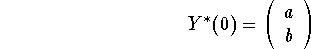,

the uniqueness and existence theorem implies that in fact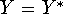gives the desired conclusion.

Remark: When you look at the above example you will notice that what made the conclusion work is that we were able to solve the algebraic systemand this was possible because the two vectors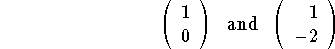are linearly independent. In fact, the above conclusion is always valid whenever we have a linear independence around.

### Theorem: The General Solution

Supposeandare two solutions to the linear system.

Assume that the vectors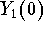and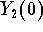are linearly independent. Then, the solution to the IVP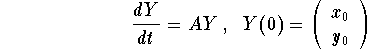,

is given by,

for some constantsand. In this case, the two-parameter family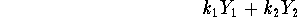,

whereandare arbitrary constants, is called the general solution of the system. Then, the two solutionsandare said to be linearly independent.

Example: Consider the undamped harmonic oscillator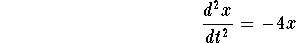.

Show that any solution x is given by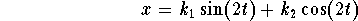.

Answer: Consider the associated linear system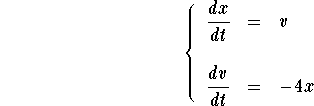Set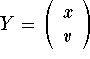. Note that the second component is just the derivative of the first one. Consider the two vector functions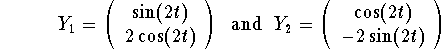It is easy to check that these two vector functions are in fact solutions to the given system. Also, you may check that the two vectors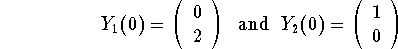are linearly independent. Therefore, any solution Y of the system is given by,

whereandare two constants. Using the first component of Y, we see that any solution x(t) of the equation is given by,

whereandare two arbitrary constants.

Clearly, the main problem now is how to find the two linearly independent solutions. This problem will be discussed in the next section and will use the eigenvalue and eigenvector technique.[Differential Equations] [First Order D.E.]
[Geometry] [Algebra] [Trigonometry ]
[Calculus] [Complex Variables] [Matrix Algebra]S.O.S MATHematics home page

Do you need more help? Please post your question on our S.O.S. Mathematics CyberBoard.Author: Mohamed Amine Khamsi